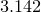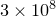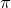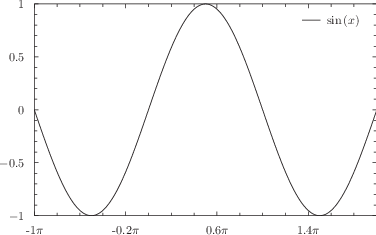## 8.8.8 Configuring how tick marks are labelled

By default, the major tick marks along axes are labelled with representations of the values represented at each point, each accurate to the number of significant figures specified using the set numerics sigfig command. These labels may appear as decimals, such as, in scientific notion, as in, or, on logarithmic axes where a base has been specified for the logarithms, using syntax such as1

set log x1 2


in a format such as.

The set xformat command – together with its companions such as set yformat2 – is used to manually specify an explicit format for the axis labels to take, as demonstrated by the following pair of examples:

set xformat "%.2f"%(x)
set yformat "%s$^\prime$"%(y/unit(feet))


The first example specifies that ordinate values should be displayed to two decimal places along the x-axis; the second specifies that distances should be displayed in feet along the y-axis. Note that the dummy variable used to represent the ordinate value is x, y or z depending on the direction of the axis, but that the dummy variable used in the set x2format command is still x. The following pair of examples both have the equivalent effect of returning the x2-axis to its default system of tick labels:

set x2format auto
set x2format "%s"%(x)


The following example specifies that ordinate values should be displayed as multiples of:

set xformat "%s$\pi$"%(x/pi)
plot [-pi:2*pi] sin(x)Note that where possible, Pyxplot intelligently changes the positions along axes where it places the ticks to reflect significant points in the chosen labelling system. The extent to which this is possible depends on the format string supplied. It is generally easier when continuous-varying numerical values are substituted into strings, rather than discretely-varying values or strings. Thus, rather thanset xformat "%d"%(floor(x))

the following is preferredset xformat "%d"%(x)

and rather thanset xformat "%s"%date.str()

the following is preferredset xformat "%d/%02d/%d"%(date.toDayOfMonth(),date.toMonthNum(), date.toYear())

### Changing the slant of axis labels

The set xformat command and its companions may also be followed by keywords which control the angle at which tick labels are drawn. By default, all tick labels are written horizontally, a behaviour which may be reproduced by issuing the command:

set xformat auto horizontal


Alternatively, tick labels may be set to be written vertically, by issuing the command

set xformat auto vertical


or to be written at any clockwise rotation angle from the horizontal using commands of the form

set xformat auto rotate 10


Axis labels may also be made to appear at arbitrary rotations using commands such as

set unit angle nodimensionless
set xlabel "I'm upside down" rotate unit(0.5*revolution)


### Removing axis tick labels

Axes may be set to have no textual labels associated with the ticks along them using the command:

set xformat ""


This is particularly useful when compiling galleries of plots using linked axes (see the next section) and the multiplot environment (see Chapter 10).

Footnotes

1. Note that the x axis must be referred to as x1 here to distinguish this statement from set log x2.
2. There is no set mxformat command since minor axis ticks are never labelled unless labels are explicitly provided for them using the syntax set mxtics (...).